# IB DP Physics: HL复习笔记10.1.4 Equipotential Surfaces

### Equipotential Surfaces

• Equipotential lines (when working in 2D) and surfaces (when working in 3D) join together points that have the same gravitational potential
• These are always:
• Perpendicular to the gravitational field lines in both radial and uniform fields
• Represented by dotted lines (unlike field lines, which are solid lines with arrows)
• In a radial field (eg. a planet), the equipotential lines:
• Are concentric circles around the planet
• Become further apart further away from the planet
• In a uniform field (eg. near the Earth's surface), the equipotential lines are:
• Horizontal straight lines
• Parallel
• Equally spaced
• Potential gradient is defined by the equipotential lines
• No work is done when moving along an equipotential line or surface, only between equipotential lines or surfaces
• This means that an object travelling along an equipotential doesn't lose or gain energy and ΔV =  0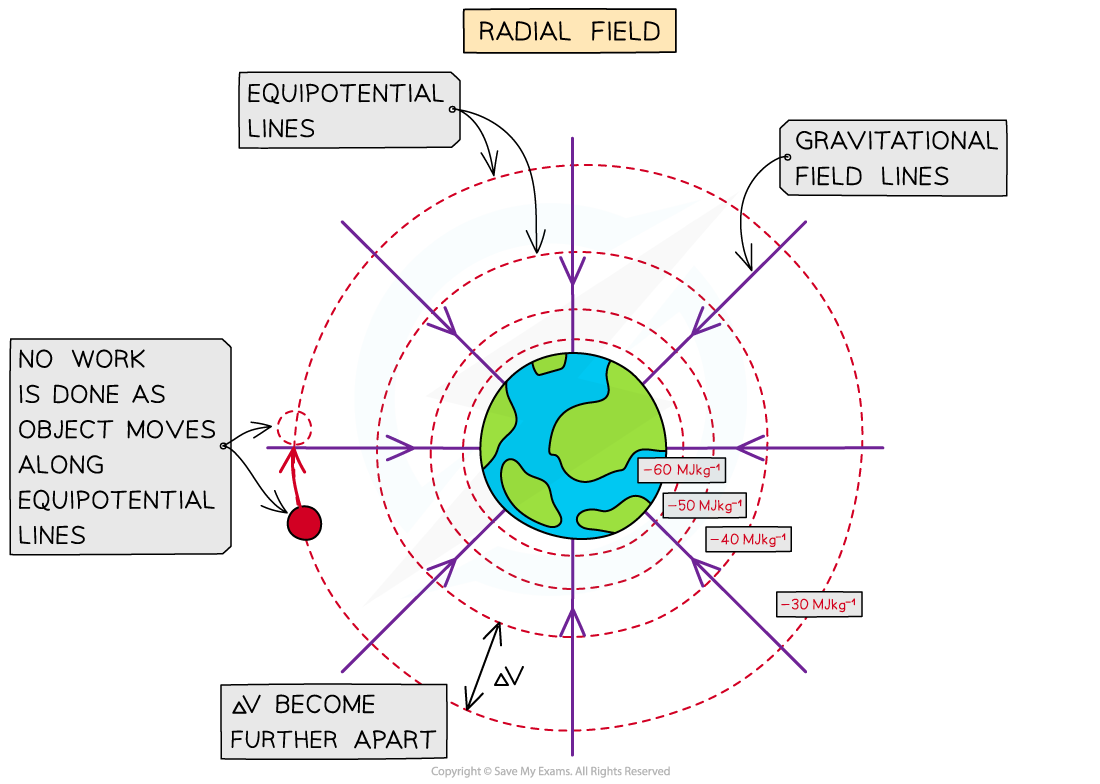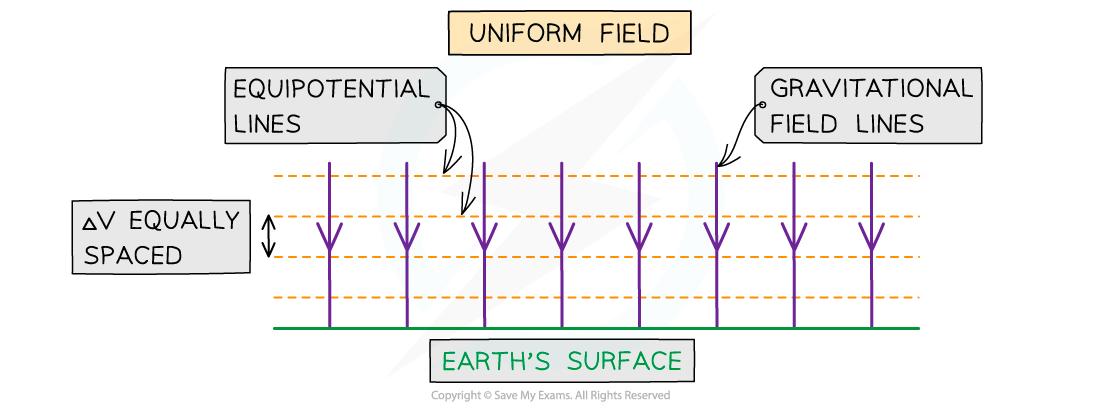Gravitational equipotential lines in a non-uniform and uniform gravitational field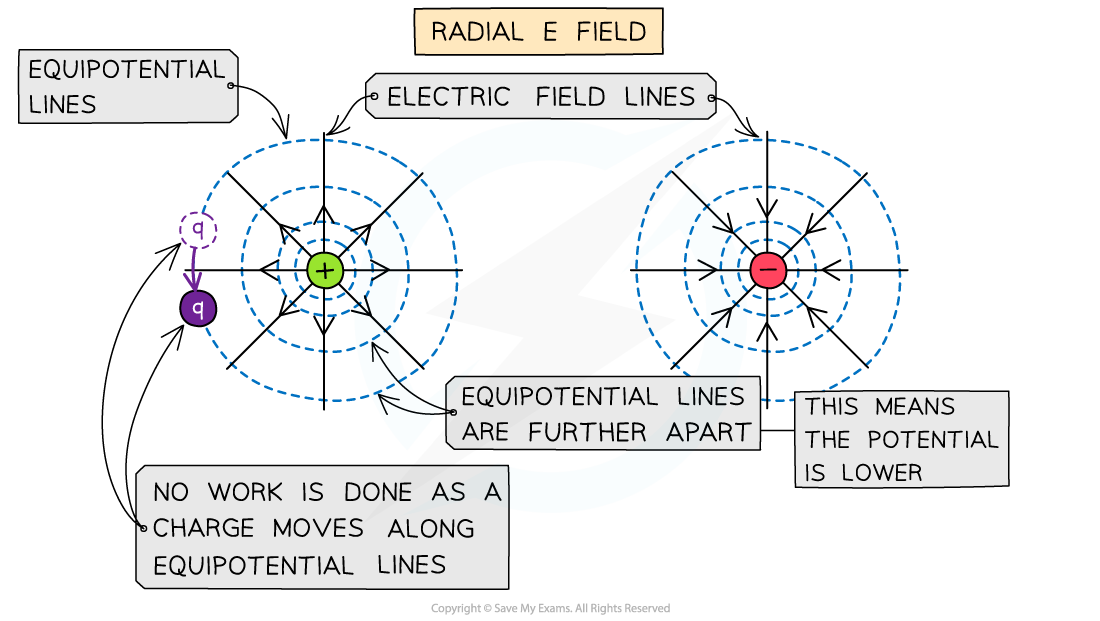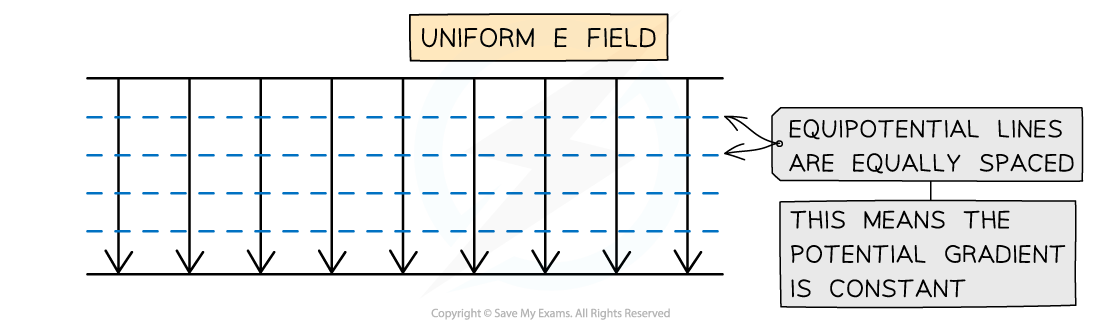Equipotential lines around a radial field or uniform field are perpendicular to the electric field lines

• The distinction between radial and uniform fields is an important one
• In a radial field (eg. a point charge), the equipotential lines:
• Are concentric circles around the charge
• Become further apart further away from the charge
• In a uniform field (eg. between charged parallel plates), the equipotential lines are:
• Horizontal straight lines
• Parallel
• Equally spaced
• Remember: uniform field is made up of lines which are a uniform distance apart

#### Worked Example

In the following diagram, two electric charges are shown which include the electric field linesa) Draw the lines of equipotential including at least four lines and at least one that encircles both charges

b) By considering the field lines and equipotentials from part (a), state what can be assumed about the two charges

Part (a)

• The lines of equipotential need to be perpendicular to the field lines at all times
• These lines are almost circular when they are near the charges
• And when moving out further the lines of equipotential cover both charges.

The lines of equipotential can be seen below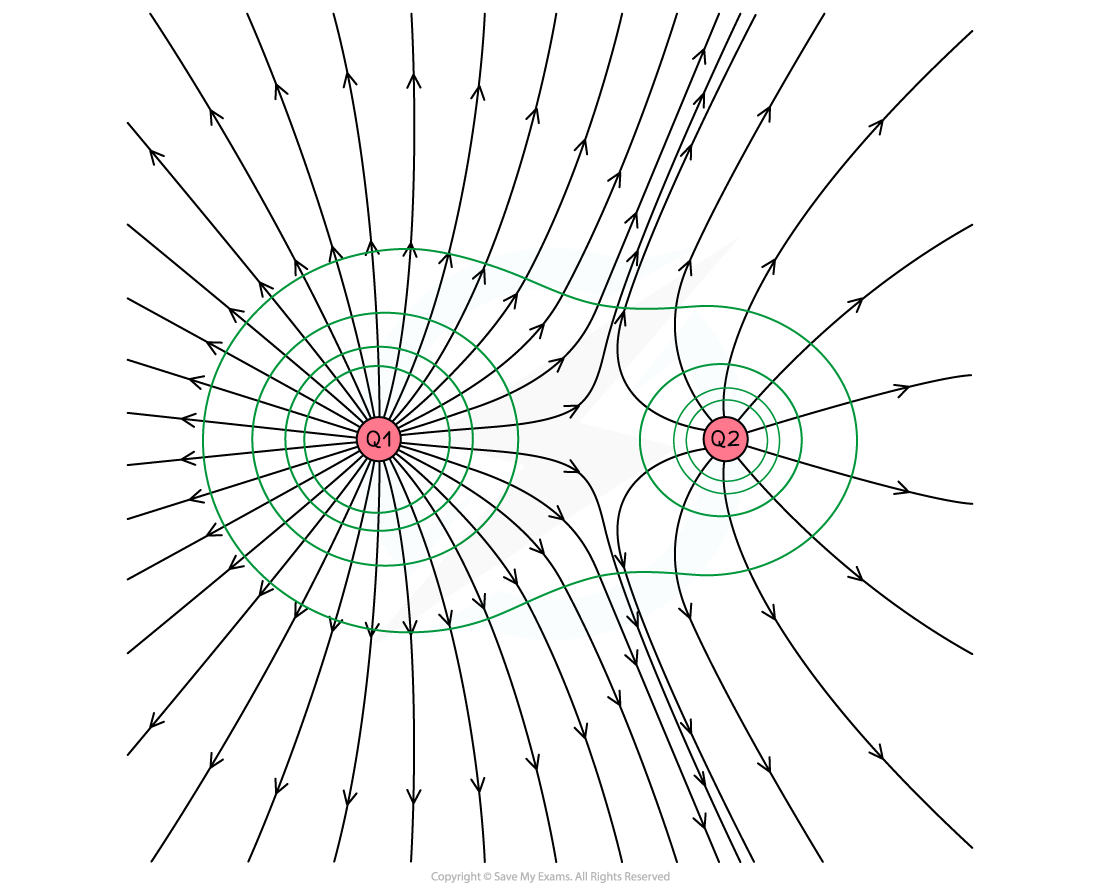Part (b)

• It can be assumed that both charges are positive since the field lines point outwards.
• It can also be assumed that the charge on the left has a larger charge than the charge on the right since:
• It has a greater density of field lines
• It has a larger sphere of influence shown by the lines of equipotential
• The point of zero electric field strength between the two charges is closer to the right charge

#### Exam Tip

Remember equipotential lines do not have arrows, since they have no particular direction and are not vectors.

Make sure to draw any straight lines with a ruler or a straight edge.